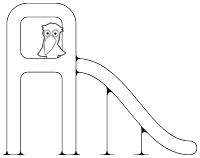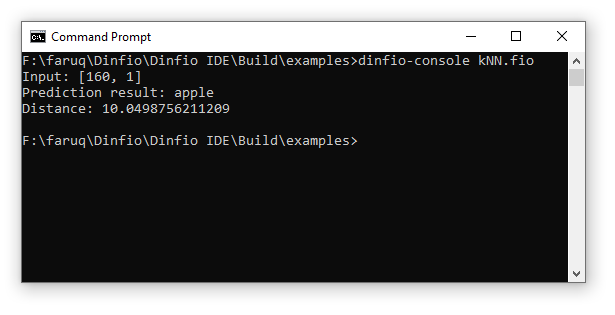### Dinfio Playground

Let's play with Dinfio!

###### Machine Learning: k-Nearest Neighbours

This is the implementation of simple k-Nearest Neighbours (kNN) classifier algorithm with Euclidean distance as the distance metric.

``````' ------------------------------------
'  Machine Learning Example in Dinfio
' ------------------------------------
'  k-Nearest Neighbours classifier
'  By: Faruq
' ------------------------------------

import math

start
var KNN: knn = KNN(3)

training_features = [
[140, 1],
[130, 1],
[150, 0],
[170, 0]
]
training_labels = ["orange", "orange", "apple", "apple"]
testing = [160, 1]

knn.train(training_features, training_labels)
prediction = knn.predict(testing)

writeln("Input: [" & testing & ", " & testing & "]")
writeln("Prediction result: " & prediction.label)
writeln("Distance: " & prediction.distance)
stop

class KNN
field x_train, y_train
field k

function construct(k)
this.k = k
stop

function train(features, labels)
this.x_train = features
this.y_train = labels
stop

function predict(test)
best_distance = this.distance(this.x_train, test)
prediction = this.y_train

for i, 1, size(this.x_train) - 1
dist = this.distance(this.x_train[i], test)

if dist < best_distance
best_distance = dist
prediction = this.y_train[i]
endif
endfor

return {label: prediction, distance: best_distance}
stop

function distance(point_1, point_2)
sum = 0

for i, 0, size(point_1) - 1
sum += (point_1[i] - point_2[i]) ^ 2
endfor

return sqrt(sum)
stop
endclass``````

Screenshot:← Back to the Dinfio Playground / Download this program